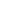Equals FunctionDetermines if two transforms are effectively the same.Syntax

 [C#] ```bool Equals(XTransform other, double epsilon) bool Equals(XTransform other) override bool Equals(object other)``` [Visual Basic] ```Function Equals(other As XTransform, epsilon As Double) As Boolean Function Equals(other As XTransform) As Boolean Overrides Function Equals(other As Object) As Boolean```Params

 Name Description other The transform to be compared against this one epsilon The largest difference in values which will still be defined as equal return Whether the two transforms are the same.Notes

 Determines if two transforms are effectively the same. Transforms contain a set of elements which are represented as floating point numbers. Floating point numbers are subject to rounding errors so the epsilon value is used to determine the resolution of the comparison. Only if two elements differ by more than the value of epsilon will they be defined as not equal. Internally Acrobat uses double precision floating point numbers for a very high level of accuracy. However within a PDF itself these numbers are typically only represented to around five decimal points. You may wish to represent similar levels of accuracy in your epsilon values. The default epsilon is zero so by default, transforms have to match perfectly for them to be considered equal.Example

 None.